×
Get Full Access to UGA - CHEM 1211 - Study Guide - Final
Get Full Access to UGA - CHEM 1211 - Study Guide - Final

×

UGA / Chemistry / CHEM 1211 / what is Stoichiometry?

# what is Stoichiometry? Description

##### Description: These notes cover all the information from the \$10 dollar review session as well as additional tips and helpful hints that will be beneficial to you on the Chem Lab I final.
11 Pages 15 Views 14 Unlocks
Reviews

Review Session: Lab Practical and Note Quiz

## what is Stoichiometry?Significant Figures

- Leading zeros are never significant, All zeros in between two nonzero  numbers are significant, Trailing Zeros are only significant if there is a  decimal point

Examples

1. 0.0201 – 3 significant figures

2. 21000- 2 significant figures

3. 30.554- 5 significant figures

4. 400.- 3 significant figures

5. 9.1 x 10-4- 2 significant figures

6. .00006- 1 significant figures

- If you are trying to measure a substance using a non-digital measuring tool  (for example: a burette), you measure one number place further than what  you can measure with certainty

- Make sure you read measurements from the tip of

the meniscus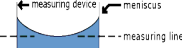## what is the equation of Percent Yield?Examples

Beaker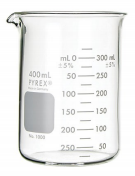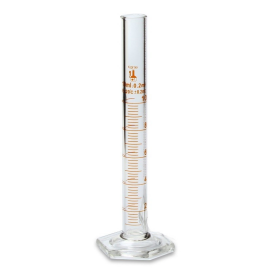We also discuss several other topics like What is Comedy?

Unlike other

equipment, a burette is read going down

## Percent Error used is used when?If you want to learn more check out What is the purpose of the trial?

You can read 21 mL Beakers are very

You can read 15.7 mL with certainty, therefore

unprecise. They can  round to the first

usually only measure to

round to second  decimal place: 21.4 mL

one significant figure: ~ decimal place: 15.75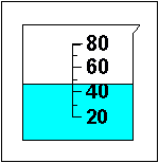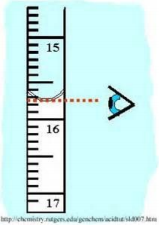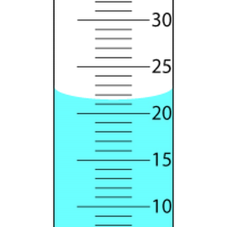We also discuss several other topics like What is the social contract?

=.8791 g = .88 g

- -

- Increasing

Precision:

Stoichiometry

- Using balanced chemical equations to compare relative amounts of reactants  and products

2 KHCO3(s) → K2CO3(s)+C O2( g )+H 2O(g)

Every reaction has a limiting reagent. If there is only one reactant in the equation than that is the limiting reagent

- Theoretical Yield (Determined by standard calculations using your starting  grams, molar mass, and mole conversion. This is what the value would be in  a perfect experiment)

Example

Starting Mass: 4.0 grams of KHC O3

4.0 g KHCO3 1 mol KHC O3 1 mol CO2 (from equation)

44.01 g CO2 (molar mass)

100.115 g

KHC O3 (molar  mass)

2 mols KHC O3 (from equation)

1molC O2

Significant Figures! Always wait until the end to  round the answer to the least significant figures  used in the problemIf you want to learn more check out What are Esteem needs?

Percent Yield

yield=experimental value

theoretical valuex 100

Example:

- The theoretical yield was determined in the previous problem (.88 g C O2 )

- The experimental yield is what you determine during your actual experiment  (For lab practical purposes, it should be given in the problem)

o Let’s say, the experiment value is .88 grams of g C O2

yield=.60 g

.88 gx 100=68.181→ 68

Percent Error

- This formula is used to measure the deviation from the theoretical value  error =|experimental value−theoretical value

theoretical value |x100

- Using the information from the previous problem

error=|(.60g)−(.88g)

(.88 g) |x 100=31.818→ 32

Experiment 1: Density- Determination of Sugar Content in  Commercial Beverages

mass(g)

Density: The ratio of a substance (

volume(cm3∨mL)

Example: You have a rectangular box with dimensions 12 cm x 20 cm x 4 cm and a  weight of 650 grams. What is the density?

V=12 cm x 20cm x 4 cm=960 c m3

D=650 g

960 c m3=.68 g /cm3

In the experiment, you first determine the densities of several known sugar  concentration solutions and use that data to make a calibration curve. Then you use the calibration curve to calculate density of an unknown solution

sugar=masssugar(g) Don't forget about the age old question of Mathematical Reasoning refers to what?

volume solvent (mL)x 100

Example:

% Sugar contains 30. Grams of sugar dissolved in 8 fl. ounces

8 fl .ounces(29.574mL

1 fl )=236.592mL

30 g

236.592mLx 100 =12.68→13 sugar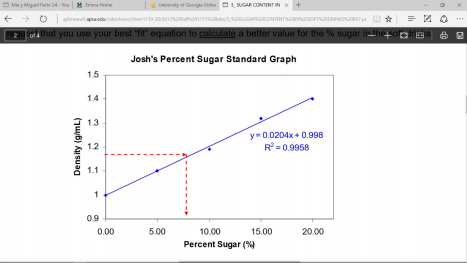If the problem ask what is the  sugar concentration at a

specific density then either use the equation (if it is given) by  plugging in the given density  as y and solving for x or see  where that specific density  touches the regression line on  the graph

R2: Just indicates linearity. The  closer the value is to 1, the

straighter the regression line is If you want to learn more check out What is the difference between heat and enthalpy?

Experiment 2: Determining Mass Percent Using Thermal  Gravimetric Analysis

In this experiment, you are finding the amount of original mass of a substance by  applying heat to decompose it

2 NaHC O3(s)→ N a2C O3(s)+C O2( g)+H2O(g)

Determine the mass loss (release of CO2 and H2O gas) from starting mass  Mass loss= Mass of CO2 + Mass of H2O

44.01 g/mol of CO2 and 18.02 g/mol of H2O= 62.03 g/ mol CO2 and H2O  *For every 2 mols of NaHCO3, you make 62.03 g/ mol of CO2 and H2O

62.03 gC O2∧H2O 1 mol NaHC O3

2 mol NaHC O384.01 g NaHC O3

(molar mass)

(Experimental Value)

=.369 mass loss/ 1 g NaHCO3 Let’s say in experiment you lose .554 g

.554 g

.369 gloss 1 g NaHC O3

→.554 g(1 g NaHCO3

.369 gloss )=1.50g NaHCO3

Experiment 3: Synthesis and Decomposition of Zinc Iodide  Decomposition by Electrolysis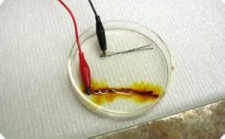Cathode (Reduction)

−¿→ Zn (s)

2+¿+2e¿

Zn¿

Red Electrode (Anode- Oxidation):  Orange/ brown color appears where  Iodide reacts

Black electrode (Cathode

Reduction): Silvery color appears  where solid Zinc decomposes

OIL RIG- Oxidation is loss of electron

Anode (Oxidation)

−¿

−¿→ I2+2e¿

2 I¿

Experiment 4: The Copper Cycle

Reaction 1- Dissolution of Copper (II) Ions

−¿ ( aq)+ N O2(s)+2 H2O(l)

2+¿ (aq)+2 N O3¿

Cu (s)+4HN O3(aq)→Cu¿

Type of Reaction: Redox (Solid Cu turns into Cu ion- it is oxidized)  Reaction 2- Formation of Cu(OH)2

CuN O3( aq)+2 NaOH ( aq) →Cu (OH )2(s)+2 NaN O3(aq)

Type of Reaction: Double Displacement and Precipitation (a solid is formed)  Reaction 3- Heating of Cu(OH)2

Cu(OH )2(s) →CuO(s)+H2O(l )

Type of Reaction: Decomposition (Heat breaks up Cu(OH)2 into two products)

Reaction 4- Formation of Cu (II) again

CuO(s)+2 H2 S O4(aq)→ CuS O4(aq)+2 H2O (l)

Type of Reaction: Double Displacement

Reaction 5a-Making Cu (s)

aq

CuS O4( aq)+Zn (s) →Cu (s)+ZnS O4¿

Type of Reaction: Redox ( Displacement

2+¿ ion∈ZnSO4( aq)−oxided

2+¿ion∈CuS O4(aq) turnsino solidCu−reduced Solid Zn turnsinto Zn¿/Cu¿

and Single

Reaction 5b- Removing excess Zn

Zn (s)+2 HCl → ZnCl2+H2(g)

Type of Reaction: Redox (Solid Zn turns into Zn2+ ion in ZnCl2( aq)−oxided ) Mass of Copper after cycle

Percent Recovery=

Mass of Copper before cyclex 100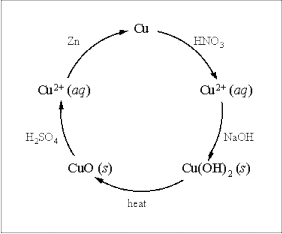Experiment 5: Separation of a Mixture

They did not go over this experiment because it required you to make your own  procedure. Just remember that you use a magnet to extract the iron, water to

dissolve the NaCl, acid to dissolve the CaCO3 that cannot be dissolved in water, and  filter out the remaining SiO2 (sand).

Experiment 6: Analysis of Vitamin C in Fruit Juices  Standardize Sodium Thiosulfate Solution

- React the Vitamin C with the known excess triiodide

- Titrate the reacted mixture of standardized sodium thiosulfate to measure  how much triiodide is leftover

- Finally, back calculate to find the amount of Vitamin C in the flask Standardize the Solution

- Titrate a known amount of triiodide in solution with a solution of sodium  thiosulfate

- Stop at the equivalence point

- At this point, all the triiodide reacted with all of the sodium thiosulfate added 2−¿

−¿+S4O6¿

2−¿→ 3I¿

−¿+2 S2O3¿

I3¿

- The amount of triiodide in the solution is exactly ½ the amount of thiosulfate  added

- The moles of thiosulfate added= 2 moles triiodide in solution -ThiosulfateConcentration ( M )=mols of thiosulfate added

Calculating Leftover Triiodide

Equivalence point is reached when  the color changes from blue to clear

- React the Vitamin C with a known excess triiodide

- Titrate reacted mixture with standardized sodium thiosulfate to measure how  much triiodide is left over

- Moles of triiodide leftover= ½ moles thiosulfate added

Calculate Vitamin C Concentration

- Moles triiodide added to solution

−¿+3H 2O

+¿→3I3¿

−¿+6 H¿

−¿+8I¿

I O3¿

- Moles triiodide added- Moles triiodide leftover = Moles of Vitamin C +¿

−¿+C6H6O6+2 H¿

−¿+C6 H8O6 →3I¿

I3¿

- Moles Vitamin C to grams Vitamin C

- Grams of Vitamin C/ fl. ounce (Juice used in the experiment= g/fl. ounce) - g/ fl. ounce = Vitamin C/ 8 ounce serving

Experiment 7: Synthesis of Alum- A Coagulant Used in  Water Treatment

In this experiment, you dissolve aluminum and react in three separate reactions to  create alum

2 Al(s)+KOH ( aq)+6H 2O (l )→ 2KAI (OH )4(aq )+3 H2(g)

2 KAI (OH) 4( aq)+H 2SO4( aq) →2 Al (OH)3(s)+2H 2O (l )+K2SO4(aq)

2 Al(OH )3(s)+3H 2 SO4( aq) → Al2 ( SO4 )3( aq)+3 H2O

*For every 2 mols of Aluminum, you make 1 mol of alum

- Test for the presence of Sulfate

Al 2( S O4)3(aq)+3Ba ( N O3)2(aq)→ 3Ba SO4(s)+2 Al( N O3)3(aq)

If a precipitation forms then there is sulfate (sulfate is not soluble in water when  reacted with barium)

- Test for presence of Aluminum (III)

+¿(aq)

3+¿ (aq)+3 N H3( aq)+3 H2O (l)+aluminon→ Al(OH )3(s)+aluminon+3 N H4¿ Al¿

If there is Aluminum (III), there will be a color change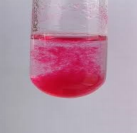Experiment 8: Spectrophotometric Analysis

In this experiment, use light to quantify a compound in solution based on absorption

- Calibrate the absorption of light vs phosphate concentration

- Measure the absorbance of lake water

- Use the curve to back calculate the phosphate concentration in the lake  water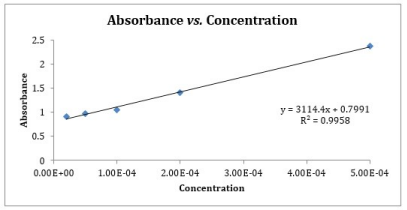The slope of the

graph (3114.4) is

used in the Lambert  Equation: A=εbc

Slope is ε

The coloring agent- compound reacts with the phosphate to make

(N H4)3P O4∙ N H4V O3∙16 MoO3

This compound absorbs strongly whereas phosphate does not

Measurement wavelength= 420 nm  (violent/blue)

Experiment 9: Calorimetry

In this experiment, you burn food below water and measure the temperature rise of  the water

q=mS∆ T

You can calculate the energy of the food sample by

masslost

Energy=heat givenoff

¿burning ¿

Experiment 10: Partial Pressure

Pcarbon dioxide=Patm−Pwater vapor−∆ h

13.6

Know how to use and spell a

Eudiometer:

A laboratory device that measures the  change in volume of a gas mixture

following a physical or chemical change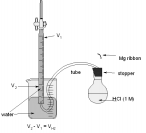What to expect!

There will be a notebook quiz and a lab practical. The note book quiz will allow you  to only use the blue graph pages from the back of your lab book so take as many  notes as possible covering the prelab, explanations, calculations, specific  observations, etc. These questions are more likely to ask about how to solve mass  percent and what color was observed during a particular reaction.

The lab practical will NOT allow you to use your notebook so make sure you  understand all the procedures to the experiments as well as the name and proper  use of all equipment.

GOOD LUCK!!!:)

Page ExpiredIt looks like your free minutes have expired! Lucky for you we have all the content you need, just sign up here